# Using the Hall Effect for Nano Electronics

The Hall effect was discovered by Edwin Hall in 1879 and has since been extensively applied in measurements, especially in materials sensing and characterization. Currently, it is being used to characterize new materials. This empowers the discovery of new phenomena, for example the spin Hall effect, the quantum Hall effect and, most recently, topological insulators1.

The Hall effect also operates as a platform for multiple device applications, for example metrology, sensing of current in the automotive industry, security screening applications and non-destructive inspection and testing.

When a conductor is placed in a magnetic field B, the Hall effect becomes relevant and this is shown in Figure 1. The charge carriers flowing through the conductor are deflected due to the Lorenz force FL and this results in a transverse, or Hall voltage Vxy, between the sides of the material at a right angle to both the current IR and the magnetic field.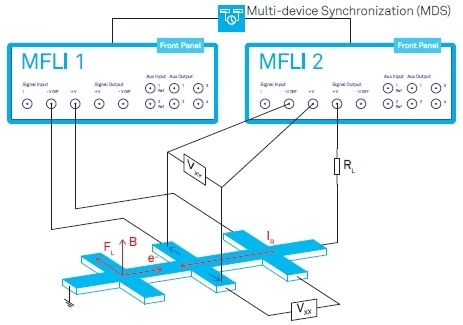Figure 1. Schematic showing the Hall effect measurement setup in a standard Hall bar geometry. Two lock-in amplifiers are used to measure the voltages Vxx and Vxy along and perpendicular to the current flow. The conventional current IR flow direction is used in the diagram, so that the motion of electrons is in the opposite direction. The Lorenz force FL is perpendicular to the magnetic field B and the charge carrier motion.

The longitudinal voltage Vxx is independent of the magnetic field whilst the relationship between the magnetic field and the Hall voltage is linearly proportional. These are the typical signatures of the Hall effect for metallic semiconductors and conductors and are shown in Figure 2(a).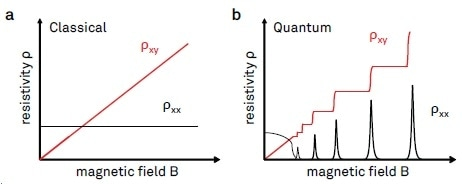Figure 2. Illustration of longitudinal and transverse resistivities ρxx and ρxy plotted as a function of the magnetic field. (a) Classical Hall effect behavior, where ρxy is co-linear with B, and ρxx is independent of B. (b) Typical signatures of the integer quantum Hall effect. The Hall resistivity ρxy shows plateaus for a range of magnetic field values, with ρxx going to zero at the same time.

The resistivity p of a bulk conductor is calculated by p = R w t/l, where w is the width, l is the length of the conductor, t is the thickness and R=V/I is the resistance. Equation 1(a) and 1(b) show the how the resistives pxx and pxy are derived from the classical Drude model:

Equation 1(a):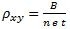Equation 1(b):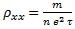In these equations, n is the density of charge carriers, e is the electron charge, τ is the mean free scattering time and m is the carrier effective mass2. The transverse Hall resistivity with a linear magnetic field and inverse density dependence is defined by Equation 1(a). The longitudinal resistivity which is independent of the field and describes the transport characteristics of the material in the direction of current is shown by Equation 1(b). The classical Hall effect has two primary applications, discussed below:

## Materials Characterization

Hall effect measurements are used to decide the conducting properties of a material. The magnetic field dependence is linear so Equation 1(a) is used to determine carrier concentration n and carrier type. Equation 1(b) is used to calculate carrier mobility carrier mobility µ= σxx/n and conductivity σxx=1/ρxx as well as longitudinal resistivity measurements. The Hall bar geometry as designed to directly extract the voltages Vxx and Vxy and the current IR is shown in Figure 1.

## Sensing

The Hall voltage and its linear proportionality to the perpendicular magnetic field component can be employed to build accurate and sensitive magnetic field sensors after the conducting properties of a material have been recognized.

In this instance, there is a proportional relationship between the magnetic field and the measured voltage and so can be determined from Equation 1(a). Hall sensors are commonly used in industrial applications in the form of speedometers, Hall probes, Hall switches, current and proximity sensors because the linearity spans from zero to fields of numerous Tesla (T).

Measuring speed is also important in a variety of these applications. Additionally, the signals are often on top of another big signal and also has to contend with background noise from various static and time varying field noise sources. These factors mean that electronic measurements can be difficult.

AC measurement techniques are especially appropriate for these situations because they can help to avoid certain mistakes in systematic measurement for example undesired components in the background noise spectrum, thermal drift and thermal offsets.

Moreover, compared to DC techniques, AC can reach and elevated signal-to-noise ratio (SNR) due mostly to the noise of the background falling as 1/f as the frequency f increases. Typically, higher SNR leads to quicker measurements. Furthermore, AC techniques often allow a larger dynamic range because they frequently come with elevated measurement resolution.

## Lock-In Amplifiers for Accurate and Quick Measurements

It is best to perform AC measurements with lock-in amplifiers. This is because they reliably measure down to a few nV with modifiable bandwidth, even when there is substantial background noise. Instruments such as this utilize phase-sensitive detection for amplitude measurements of a signal and the phase against a frequency of reference. Aside from the outside the measurement bandwidth and the reference frequency, background noises do not affect the measurement because they are heavily rejected.

A classic setup utilizing the Hall bar geometry and two Zurich Instruments MFLIs, 500 kHz lock-in amplifiers, is shown in Figure 1. Both of the lock-in amplifiers are operated to measure the longitudinal voltage Vxx and the transverse Hall voltage Vxy while one of the two supplies the current through the sample through a current limiting resistor RL.

To enable the assumption that the current is constant, this kind of resistor is usually selected to be much bigger than any of the resistances combined in the circuit. For characterization of the materials, frequencies of up to a few tens of Hz are usually used, with the two lock-in amplifiers coordinated with regards to data sampling and measurement frequency.

Although the level current assumption is true in many cases, in experiments where the sample impendence changes dramatically over the course of a measurement, the current needs to be carefully monitored. The MFLI is able to do this using its current sensing input which records the current at the same frequency as the voltage input. More detailed treatment of lock-in amplifiers and how they function is provided in Source 3.

## Quantum Hall Effects in 2DEGs

When the electrons of a two-dimensional electron gas (2DEG) are put in a magnetic field, the Hall effect shows qualitatively new features. As the magnetic field increases, the Hall resistivity alters in steps and plateau structures form. At the same time, the longitudinal resistivity drops to zero. This is a quantum mechanical phenomenon whereby the discrete energy levels, called Landau levels, are developed in the density states of a 2DEG. This is called the quantum Hall Effect (QHE).

The classic behavior is shown in Figure 2(b). The electrons on a plateau move macroscopic distances following the edges of a sample with no resistance and follow distinct circular orbits with quantized energy levels. This is remarkable as the conduction remains carried by the electrons and the dissipation-less nature indicate the topologically protected edge states.

Moreover, visible “quantum oscillations” called Shubnikov-de Haas (SdH) oscillations, can be caused by the electrons moving from one Landau level to another, as shown in Figure 3. Klaus von Klitzing earned the Nobel prize in 1985 for his discovery of the integer QHE in 19804.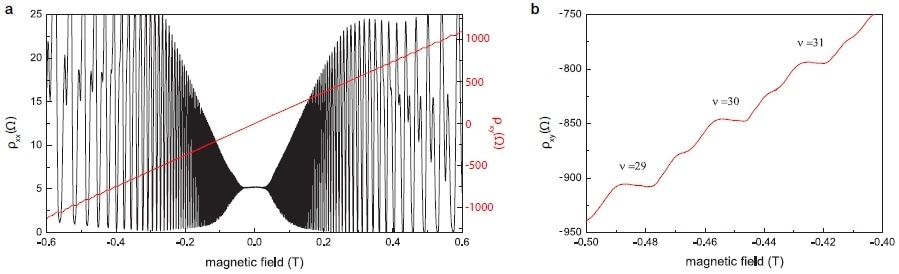Figure 3. (a) Plot of longitudinal and transverse resistivity ρxx and ρxy as a function of the magnetic field B, using two lock-in amplifiers. Note that ρxychanges sign when the field direction is inverted. (b) A zoom into the measurement data from (a)shows several higher order Hall plateaus at negative fields with the prominent signatures of spin splitting between them.

Equation 2 gives the Hall resistivity for a specific plateau:

Equation 2: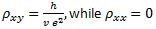In this equation, h/e2 is a fundamental constant called the von Klitzing constant equal to RK=25813.807557(18)Ω since ρ = R w t/l and v is an integer called the filling factor. The RK depends on the electron charge e and the Planck constant h and not on temperature fluctuations, crystal impurities or material properties.

Other forms of Hall effect phenomena have been found in addition to the QHE in a 2DEG. These include the spin Hall effect, the fractional Hall effect and research has also revealed a “quantum anomalous Hall effect.” This is where topological insulators as thin films are able to exhibit a QHE at zero external magnetic field5.

## Hall Effect Case Study

The QHE was measured at the ETH Zurich where 2DEG GaAs/Al0.3Ga0.7 using two MFLIs. Samples were characterized for the intention of a study of light-matter coupling6. The density of electrons was 3x1011 cm-2 and the electron mobility µ was 3x106 cm2 /V s. The dimensions of the Hall bar were 39 µm width and 166.5 µm length.

An example of measurements, performed at a frequency of 14 Hz and at 100 mK are shown in Figure 3. Applying a RMS voltage of 200 mV across the 10 MW current limiting resistor, which was placed in a series with a Hall bar, attainted a current of 20 nA. Using a home-built preamplifier with a signal amplification of 1,000 before the lock-in point achieved signal amplification. A time constant of 100 ms was used to carry out the lock-in measurements.

Figure 3 shows the longitudinal and transverse resistivities Pxx and Pxy as a function of the magnetic field B. They were found using two lock-in amplifiers. The black trace shows SdH oscillations in Pxx and the red trace shows the integer quantum Hall effect plateaus in pxy. The first plateau develops at about 13 T and is no displayed. A filling factor of as much as 300 was seen in this measurement, with high sample mobility and low temperatures causing early onset of SdH oscillations. This reflects the high quality of the sample. Structures in Pxx that develop above 0.4 T are due to the spin splitting of the Landau levels and can be seen n Figure 3(b).

## Practical Uses of QHE and Related Phenomena

2DEG materials are employed as resistance standards because the resistance of the quantum Hall states is separate from material type, temperature and scattering. Until recently, QHE was only seen at low temperatures. Graphene measurements at a magnetic field of 20 T showed the QHE at room temperature in 20077, and this is the possible basis for developing new resistance standards. Another set of new possibilities is allowed by the finding of the QHE in topological insulators that lack the presence of a magnetic field. New materials such as these that conduct in protected states could be used in fast electronics and quantum computation1.

## Advantages of Using the MFLI for Hall Effect Measurements

### High Sensitivity to Extract the Smallest Signals

Measurements of the Hall effect implicate the detection of small signals that are commonly buried in the noise. Usually, the resistance in Hall Effect Measurements is approximately 100 W or less. Combined with a current of about 20 nA, this changes into voltages of a few mV for Vxx and hundreds of mV for Vxy.

Employing a preamplifier is definitely beneficial to increase the SNR through amplification and filtering of the broadband noise. To make room for the full magnetic field sweep, both voltage measurements require the high dynamic range of the MFLI. This is also critical in order to resolve small features.

### Effective Noise Rejection to Maximize SNR

In order to attain high SNR, it is essential to effectively reject noise. The MFLI has eight order filters and these can reject noise as much as a million times larger than the signal that is measured. This delivers a big enough margin to optimize for measurement speed as well as accuracy.

Moreover, the noise coming from the lock-in input influences the electronic temperature of the sample and adds to the overall noise when measurements are conducted at low temperatures. Due to its inputs having the lowest power dissipation available which makes the MFLI the best commercial answer on the market8.

The measurement is essentially hastened by the effective noise rejection of the lock-in. This is due to the fact that the filter time constants can be shortened and the full characterization measurement time lasts from a day to a week can also be reduced, by as much as a factor of ten.

### High Accuracy: Dedicated Current Sensing

It is vital to measure the current IR to get highly accurate resistance measurements and circumvent the systematic error of as much as 10% in situations where the current IR assumption does not hold. The current can be measured simultaneously with the Hall voltage by using one lock-in amplifier unit with the MF-MD option accessible. The operator benefits from maximized measurement fidelity and minimized setup complexity.

### Efficient Work-Flows: LabOne Software Included

The Zurich Instruments control software, LabOne, is provided with the MFLI and is developed for efficient work flows. A hoard of features and tools will aid in gaining high confidence in the data collected and the instinctive operator interface quickly delivers initial results to the user.

For example, demodulator outputs can be visualized in the time (frequency) domain using the Plotter (Spectrum Analyzer) whilst data is being directly recorded at the Signal Inputs with the Scope.

If measurements necessitate utilizing more than one instrument, there is a multi-device synchronization (MDS) feature available. MDS aligns the time stamps of the recorded data and retains the reference clocks of all synchronized instruments. The measurements can be carried out during one session of the LabOne operator interface.

The LabOne offers APIs for LabVIEW®, MATLAB®, Python, .NET and C if the measurements need to be automated of the MFLI is being integrated into a pre-existing measurement setting.

## Conclusion

Currently, the Hall effect measurements are universal in SI unit redefinition, industrial applications and fundamental research. Most of the measurements benefit from using AC techniques where lock-in amplifiers are the main tool for ensuring SNR and accuracy through optimum rejection of noise.

The MFLI Lock-In Amplifier from Zurich Instruments is developed on the latest software and hardware technologies and unites the benefits of easy use and high performance digital signal processing.

MFLI is the ideal tool for a variety of applications: from simple measurements to detect a Hall voltage at a defined frequency to more complicated setups that require the use of multiple instruments. It can adapt to changing demands, for example adding another 3 demodulators to analyze the voltage and current inputs at the same time and upgrading the frequency range from DC to 500 kHz to DC to 5 MHz.

## References

1. Davide Castelvecchi. The strange topology that is reshaping physics. Nature, 547:272–274, 2017.
2. N. W Ashcroft and N.D. Mermin. Solid state physics. 1976.
3. Zurich Instruments AG. Principles of Lock-in Detection, 2017. White Paper.
4. K. v. Klitzing, G. Dorda, and M. Pepper. New method for high-accuracy determination of the fine-structure constant based on quantized hall resistance. Phys. Rev. Lett., 45:494–497, Aug 1980.
5. A. J Bestwick, E. J. Fox, X. Kou, L. Pan, K. L. Wang, and D. Goldhaber-Gordon. Precise quantization of the anomalous hall effect near zero magnetic field. Phys. Rev. Lett., 114:187201, May 2015.
6. G. L. Paravicini-Bagliani, F. Appugliese, E. Richter, F. Valmorra, J. Keller, M. Beck, N. Bartolo, C. Rössler and T. Ihn, K. Ensslin, C. Ciuti, G. Scalari, and J. Faist. Magneto-transport controlled by landau polariton states. arXiv:1805.00846, 2018.
7. K. S. Novoselov, Z. Jiang, Y. Zhang, S. V. Morozov, H. L. Stormer, U. Zeitler, J. C. Maan, G. S. Boebinger, P. Kim, and A. K. Geim. Room temperature quantum hall effect in graphene. Science, 315(5817):1379–1379, 2007.
8. Zurich Instruments AG. Power Dissipation at Input Connectors of Lock-in Amplifiers, 2017. Technical Note.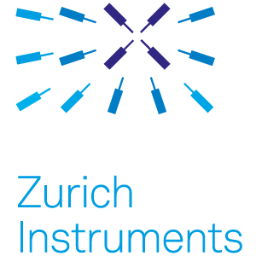This information has been sourced, reviewed and adapted from materials provided by Zurich Instruments.

## Citations

• APA

Zurich Instruments AG. (2019, March 28). Using the Hall Effect for Nano Electronics. AZoNano. Retrieved on June 05, 2020 from https://www.azonano.com/article.aspx?ArticleID=5177.

• MLA

Zurich Instruments AG. "Using the Hall Effect for Nano Electronics". AZoNano. 05 June 2020. <https://www.azonano.com/article.aspx?ArticleID=5177>.

• Chicago

Zurich Instruments AG. "Using the Hall Effect for Nano Electronics". AZoNano. https://www.azonano.com/article.aspx?ArticleID=5177. (accessed June 05, 2020).

• Harvard

Zurich Instruments AG. 2019. Using the Hall Effect for Nano Electronics. AZoNano, viewed 05 June 2020, https://www.azonano.com/article.aspx?ArticleID=5177.